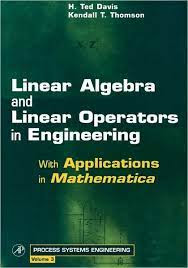ALGEBRA MATHEMATICS

# Linear Algebra and Linear Operators in Engineering With Applications in Mathematica Vol 3 Free DownloadDesigned for advanced engineering, physical science, and applied mathematics students, this innovative textbook is an introduction to both the theory and practical application of linear algebra and functional analysis. The book is self-contained, beginning with elementary principles, basic concepts, and definitions. The important theorems of the subject are covered and effective application tools are developed, working up to a thorough treatment of eigenanalysis and the spectral resolution theorem. Building on a fundamental understanding of finite vector spaces, infinite dimensional Hilbert spaces are introduced from analogy. Wherever possible, theorems and definitions from matrix theory are called upon to drive the analogy home. The result is a clear and intuitive segue to functional analysis, culminating in a practical introduction to the functional theory of integral and differential operators.